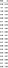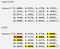# Link to Library: https://github.com/yang-zhang/product_category

Product category prediction model built with:

and trained using Amazon product data.

This library supports

• Predicting categories using the pretrained model.
• Training from scratch, with a transformers model as the starting point.
• Transfer learning from the pretrained model.

# Pretrained model

The pretrained model is trained using product category and title in the metadata Amazon product data. Each product can have multiple categories. We sample 500K products (85% for train; 15% for validation) to train the model, which resulted in ~1900 categories. We use pytorch-lightning to train a multilabel classification model with the pretrained model from huggingface/transformers as the…

# Pytorch Lightning for 🤗 Huggingface Transformers Language Modeling

Language Modeling Example with Pytorch Lightning and 🤗 Huggingface Transformers.

Language modeling fine-tuning adapts a pre-trained language model to a new domain and benefits downstream tasks such as classification. The script here applies to fine-tuning masked language modeling (MLM) models include ALBERT, BERT, DistilBERT and RoBERTa, on a text dataset. Details about the models can be found in Transformers model summary.

The Transformers part of the code is adapted from examples/language-modeling/run_mlm.py. Finetuning causal language modeling (CLM) models can be done in a similar way, following run_clm.py.

PyTorch Lightning is “The lightweight PyTorch wrapper for high-performance AI research. Scale your models…

# LaBSE Pytorch Model

I’ve converted LaBSE model weights to Pytorch model weight and shared on https://github.com/yang-zhang/labse-pytorch

LaBSE is from Language-agnostic BERT Sentence Embedding by Fangxiaoyu Feng, Yinfei Yang, Daniel Cer, Naveen Arivazhagan, and Wei Wang of Google AI.

# Abstract from the paper

We adapt multilingual BERT to produce language-agnostic sen- tence embeddings for 109 languages. While English sentence embeddings have been obtained by fine-tuning a pretrained BERT model, such models have not been applied to multilingual sentence embeddings. Our model combines masked language model (MLM) and translation language model (TLM) pretraining with a translation ranking task using bi-directional dual encoders. The resulting multilingual sentence embeddings improve average…

# Explain Pytorch Tensor.stride and Tensor.storage with code examples

Pytorch’s class has a and a method. They are not very often used directly, but can sometimes be helpful when you need to take a closer look at the underlying data. (I’ll show an example of using them to illustrate the difference between and at the end.)

As explained in Pytorch’s document, simply “returns the underlying storage”, which is relatively straightforward.

But the explanation for from Pytorch’s document a bit difficult for me to understand:

Each strided tensor has an associated , which holds its data. These tensors provide multi-dimensional, strided view of…

# The “gradient” argument in Pytorch’s “backward” function — explained by examples

This post is some examples for the argument in Pytorch's function. The math of is explained in this tutorialand these threads (thread-1, thread-2), along with some examples. Those were very helpful, but I wish there were more examples on how the numbers in the example correspond to the math, to help me more easily understand. I could not find many such examples so I will make some and write them here, so that I can look back when I forget this in two weeks.

In the examples, I run code in torch, write down the math, and…

# Table of cross-entropy loss at different probabilitiescross-entropy loss at different probabilities for the correct class

Cross-entropy loss is used for classification machine learning models. Often, as the machine learning model is being trained, the average value of this loss is printed on the screen. But it is not always obvious how good the model is doing from the looking at this value.

The formula of cross entropy in Python is

`def cross_entropy(p):    return -np.log(p)`

where is the probability the model guesses for the correct class.

For example, for a model that classifies images as an apple, an orange, or an onion, if the image is an apple and the model predicts probabilities {“apple”: 0.7…

# Setup docker for Kaggle

Here are some notes on setting up docker for Kaggle (especially on installing and enabling nbextensions). I had to do this from time to time and wanted to write the steps down for the record. I put it here in case it’s useful for someone else.

# Why use docker for Kaggle

Kaggle is a good place to learn machine learning and data science. I think its docker image is a good option for data science development environment for two scenarios:

# Scaling in Neural Network Dropout Layers (with Pytorch code example)Scaling in dropout

For several times I confused myself over how and why a dropout layer scales its input. I’m writing down some notes before I forget again.

Link to Jupyter notebook:

In Pytorch doc it says:

Furthermore, the outputs are scaled by a factor of 1/(1-p) during training. This means that during evaluation the module simply computes an identity function.

So how is this done and why? Let’s look at some code in Pytorch.

Create a dropout layer with a dropout rate :

`import torchimport numpy as npp = 0.4m = torch.nn.Dropout(p)`

As explained in Pytorch doc:

# Multi-task Deep Learning Experiment using fastai Pytorch

This post is an abstract of a Jupyter notebook containing a line-by-line example of a multi-task deep learning model, implemented using the fastai v1 library for PyTorch. This model takes in an image of a human face and predicts their gender, race, and age.

The notebook wants to show:

1. an example of a multi-task deep learning model;
2. the multi-task model makes better predictions than the individual model; and
3. how to use the fastai library to easily implement the model.

The Jupyter notebook is working and runnable, so you can run and change the code if you like (at least it’s…

# Multi-label classification example using fastai v1

Simple example of multi-label classification using fastai v1.

`%reload_ext autoreload%autoreload 2from fastai import *from fastai.vision import *`

In :

`path = untar_data(URLs.PLANET_SAMPLE)path`

Out:

`PosixPath('/home/ubuntu/.fastai/data/planet_sample')`

In :

`data = ImageDataBunch.from_csv(path, folder='train', sep=' ', suffix='.jpg', ds_tfms=get_transforms(), tfms=imagenet_norm, size=224)img,labels = data.valid_ds[-1]img.show(title=" ".join(np.array(data.classes)[labels.astype(bool)]))`

Train last layer:

`learn = ConvLearner(data, models.resnet34, metrics=Fbeta(beta=2))learn.fit_one_cycle(1)Total time: 00:10epoch  train loss  valid loss  fbeta   1      0.748020    0.649659    0.365789  (00:10)`

Unfreeze and finetune:

`learn.unfreeze()learn.fit_one_cycle(1)Total time: 00:10epoch  train loss  valid loss  fbeta   1      0.621923    0.705165    0.380751  (00:10)`## Yang Zhang

Software Engineering SMTS at Salesforce Commerce Cloud Einstein